# Basic model building and training#

Examples introducing fundamental model building and training strategies.Simple training on MNE epochs

Simple training on MNE epochs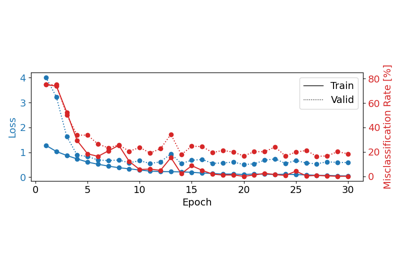Cropped Decoding on BCIC IV 2a Dataset

Cropped Decoding on BCIC IV 2a Dataset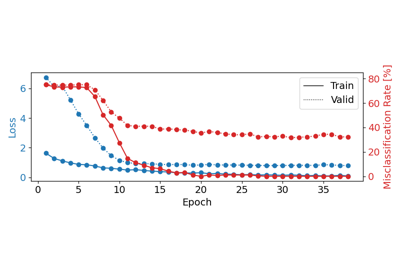Basic Brain Decoding on EEG Data

Basic Brain Decoding on EEG Data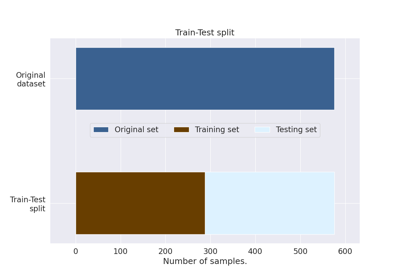How to train, test and tune your model?

How to train, test and tune your model?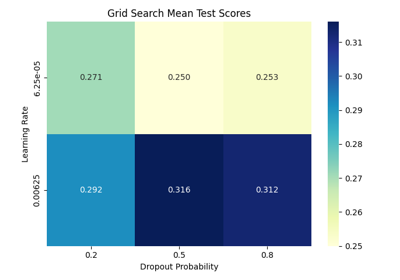Hyperparameter tuning with scikit-learn

Hyperparameter tuning with scikit-learn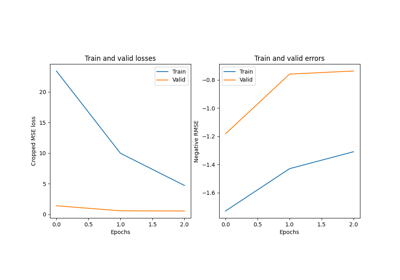Convolutional neural network regression model on fake data.

Convolutional neural network regression model on fake data.Training a Braindecode model in PyTorch

Training a Braindecode model in PyTorch